Introduction

The goal of this package is to provide a simple workflow for a customized graph customized to your need.

Setup

library(survival)
library(mstate)
library(survCurve)

The basic principle

Most available function for plotting Kaplan Meier estimator or cumulative incidence have a main plot function which does all the work “magically”. They have a syntax like this:

plot(model,options)

Although these functions usually allow some customization, often you can not format the graph as you actually wish. The plot function in this package have a different idea. First you create an empty plot surface, then you have to create each element of the graph separately.

survPlot(options)
confIntArea(model,group,event,options)
confIntArea(model,group,event,options)
survCurve(model,group,event,options)
survCurve(model,group,event,options)
nrAtRisk(model,group,options)
nrAtRisk(model,group,options)

This approach takes considerable more code, however you can stack and mix the elements easier, and you can combine them from different models. Furthermore, you can easier change the option of a single element. The first function does not take the model as an argument. therefore, it requires at least a minimum of information on the plot size (at least xmax). The other function work independently of the survPlot option, and independently of each other. They can also be used on top of an other plot (comparable to the functions “line()”, “segements()”, “text()”, etc. of the core package). Also, none of the functions does write any variable into the global environment. The number in competitive risk models do not different for the events, thus the function “nrAtRisk()” does not have “event” as an argument.

Simple use cases

The functions defaults is usually covers the most likely application. Also the group (equals to strata) can be omitted if the model has only one group. If the model is a Kaplan Maier model, the event number can be omitted. Depending on the model, the code can be much less, for a simple Kaplan Meier graph with no groups, the code is like:

survPlot(options)
confIntArea(model,options)
survCurve(model,options)
nrAtRisk(model,options)

Supported models

The model can be a survfit object (survival-package) or a cuminc object (mstate-package). cumminc objects form the cmrsk packages are currently not supported.

The empty plot, and the number at at risk

The package is one of the few package which does provide automated plotting of the number at risk. By default the function plots the numbers at y=0.08, which is usually just above the x-axis. Depending on your graph, or if you have different groups, you can easy adjust that value. The survPlot functions support to have some extra space below the plot, which is the “space.nrAtRisk” option. At default, the space.nrAtRisk is 0, thus this space is omitted. The space.nrAtRisk is actually inside the plot. Therefore, it is not dependant to graphical options “mar”, oma" etc. of the par() option. ymax and ymin are 1.02 and -0.02. defaut for xmin is 0, xmax must be specified. At Default, the y-Axis is scaled by 100, because usually survival and cumulative incidence are communicated as percentage. One has to remember that the actual numbers on the plot region are 100 times smaller as on the axis (e.g. 0 to 100 on the y-axis but 0 to 1 in the actual plot).

survPlot(xmax=10,space.nrAtRisk=0.2)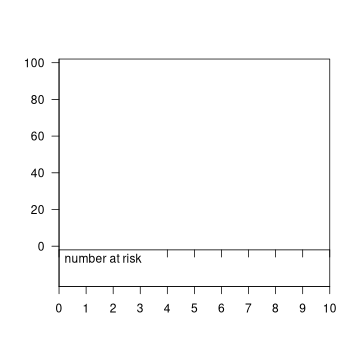The nr at risk can be labeled with a small marker (flag) at the right of the plot, so it is possible to identify to which curve of the plot they belong. If inside the number at risk function, one of the options “bgcol.flag”,“lncol.flag” or “lty.flag” is specified, the flag is drawn. The “label” adds a label behind the flag.

survPlot(xmax=..., space.nrAtRisk=0.35, ...)
survCurve(model, group=1 lty=1, ...)
survCurve(model, group=2 lty=2, ...)
nrAtRisk(model, y=-0.15, group=1, lty.flag=1, label="Group A", ...)
nrAtRisk(model, y=-0.25, group=2, lty.flag=2, label="Group B", ...)

If you want to label the curves in a similar way independently to the nrAtRisk() function, there is the “survLable” function which does that.

Suggested use

I suggest the following use

1. Define transparent color for the confidence intervalls
2. Make an empty plot with survPlot
3. Draw all the confidence intervals.
4. Draw the lines, so they come on top on the confidence intervalls
5. Draw the number at risk

Of course you always have to tweak the graph a little bit. Also, the result may depend on the size of your output device. For details, see the documentation of the functions (?survPlot, ?survCurve, ?confIntArea, ?nrAtRisk).

Examples

In the following, there are examples using the sample-data from the “mstate” package and the “survival” package.

A very common Kaplan Maier scenario

model <- survfit(Surv(aml\$time,aml\$status)~aml\$x)

survPlot(main="Survival- AML",
xmax=50,
space.nrAtRisk=0.20,
title.xaxis="months after event",
title.yaxis="                   (%)",
title.nrAtRisk="patients at risk",
font.xaxis=2, font.yaxis=2)

confIntArea(model, col=col1, group=1)
confIntArea(model, col=col2, group=2)

survCurve(model, lty=1, group=1)
survCurve(model, lty=2, group=2)

nrAtRisk(model, group=1,
label="Maint.",
bgcol.flag=col1,
lty=1,
ypos=-0.10)
nrAtRisk(model, group=2,
label="non-Maint.",
bgcol.flag=col2,
lty=2,
ypos=-0.17)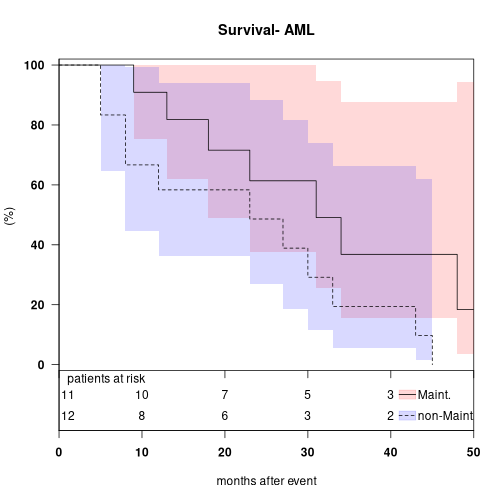A very simple Kaplan Maier scenario

model <- survfit(Surv(aml\$time,aml\$status)~0)

col <- "grey"

survPlot(main="Survival- AML",
xmax=55,
title.xaxis="months after event",
title.yaxis="                   (%)"
)

confIntArea(model, col=col)

survCurve(model)

nrAtRisk(model)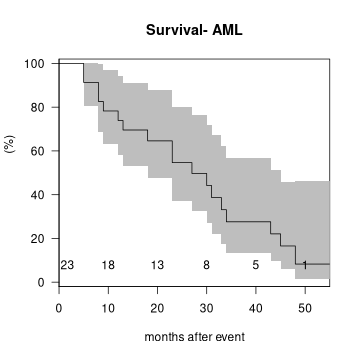A common cumulative incidence scenario

data(aidssi)
model <- Cuminc(time="time", status= "status", failcodes=c(1,2), data=aidssi)

survPlot(main="Disease progression after HIV-infection",
xmax=14,
ymax=0.65,
space.nrAtRisk=0.12,
title.xaxis="years after hiv-infection",
title.yaxis="               incidence (%)",
title.nrAtRisk="patients at risk",
font.xaxis=2, font.yaxis=2)

confIntArea(model, col=col1, event=1)
confIntArea(model, col=col2, event=2)

survCurve(model, event=1, lty=3)
survCurve(model, event=2, lty=2)

nrAtRisk(model, ypos=-0.1,cex.nr=0.8)

survLable(x=1, y=0.6, text="AIDS",bgcol.flag=col1,lty=3)
survLable(x=1, y=0.50, text="SI-Apperance",bgcol.flag=col2,lty=2)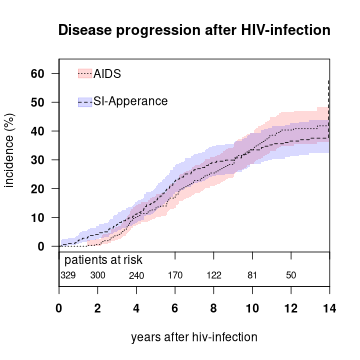A complex, but also common cumulative incidence scenario

data(aidssi)
par_backup <- par(mfrow=c(1,2))
model2 <- Cuminc(time="time", status= "status", group="ccr5", failcodes=c(1,2), data=aidssi)

survPlot(main="ccr5 ww\n(2x wild allele)",
xmax=14,
ymax=0.65,
space.nrAtRisk=0.12,
title.xaxis="years after hiv-infection",
title.yaxis="               incidence (%)",
title.nrAtRisk="patients at risk",
font.xaxis=2, font.yaxis=2)
confIntArea(model2, col=col1, group=1, event=1)
confIntArea(model2, col=col2, group=1, event=2)
survCurve(model2, group=1, event=1, lty=3)
survCurve(model2, group=1, event=2, lty=2)
nrAtRisk(model2, group=1, ypos=-0.1,cex.nr=0.8)
survLable(x=1, y=0.6, text="AIDS",bgcol.flag=col1,lty=3)
survLable(x=1, y=0.50, text="SI-Apperance",bgcol.flag=col2,lty=2)
#
survPlot(main="ccr5 wm\n(1 wild, 1 mutant allele )",
xmax=14,
ymax=0.65,
space.nrAtRisk=0.12,
title.xaxis="years after hiv-infection",
title.yaxis="               incidence (%)",
title.nrAtRisk="patients at risk",
font.xaxis=2, font.yaxis=2)
confIntArea(model2, col=col1, group=2, event=1)
confIntArea(model2, col=col2, group=2, event=2)
survCurve(model2, group=2, event=1, lty=3)
survCurve(model2, group=2, event=2, lty=2)
nrAtRisk(model2, group=2, ypos=-0.1,cex.nr=0.8)
survLable(x=1, y=0.6, text="AIDS",bgcol.flag=col1,lty=3)
survLable(x=1, y=0.50, text="SI-Apperance",bgcol.flag=col2,lty=2)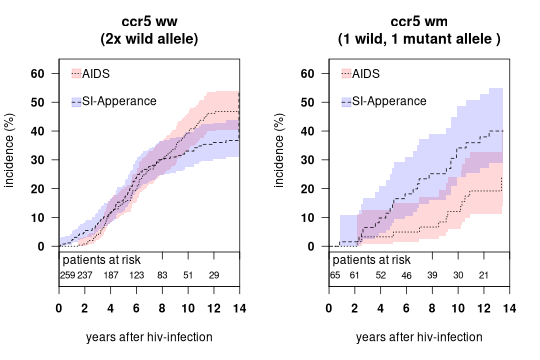par(par_backup)